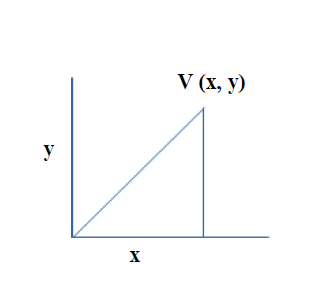# Golang program to calculate the absolute and scale of a vertex.

## ExampleAbs(x, y) => √(x)2+(y)2

Scale(f) => (x*f, y*f)

Approach

• Define a vertex as a struct.

• Initialize the vertex with some x and value.

• Define a method to calculate absolute(x, y).

• Define a method to calculate scale(x*f, y*f).

## Example

Live Demo

package main
import (
"fmt"
"math"
)
type Vertex struct {
X, Y float64
}
func Abs(v Vertex) float64{
return math.Sqrt(v.X*v.X + v.Y*v.Y)
}
func Scale(v *Vertex, f float64) {
v.X = v.X * f
v.Y = v.Y * f
}
func main() {
v := Vertex{3, 4}
fmt.Println("Given vertex is: ", v)
fmt.Println("Absolute value of given vertex is: ", Abs(v))
f := 10.0
fmt.Printf("After scaling by %0.1f new vertex is: ", f)
Scale(&v, f)
fmt.Println(v)
fmt.Println("Absolute value of given vertex is: ", Abs(v))
}

## Output

Given vertex is: {3 4}
Absolute value of given vertex is: 5
After scaling by 10.0 new vertex is: {30 40}
Absolute value of given vertex is: 50